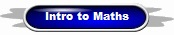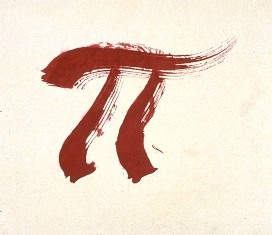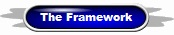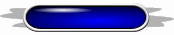MathsUseful Information What is Maths? Negative Numbers Proof by Exhaustion Why We Count to 10 The Decimal Revolution The Invention of Algebra

 Related Articles The Riemann Hypothesis The Golden Mask A Philosophy of Maths? A Knotty Problem Mathematically Challenged Mechanical Mathematicians Maths as Glue Criminal Maths The Maths of War Number Without Counting Why Maths Works 7 Important Equations Dangerous Maths Unreasonably Effective? Mathematical Beauty Maths - The Grand Design? A Biological Basis for Maths

 TED Talks Mathemagic Maths in Nature The Lost Codex Lovely Prime Numbers The Mathematics of War Super Symmetry The Mathematics of Love Maths is Forever Math & Art = Origami Sexy Maths Mathematical Perspectives Spotting Bad Statistics

 Useful Websites Axioms, Theorems and Proofs - How Maths Works The Misuse of Statistics The Growth and Development of Maths Fractals as Art Introduction to the Philosophy of Mathematics The Indispensibility of Maths to Science Math World Essays on the Philosophy of Maths Maths Essays from J.R. Lucas Maths Puzzles The History of Maths The Art of Mathematics The Philosophy of Mathematics